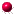XMM-Newton Science Analysis System

asmooth (asmooth-2.32.1) [xmmsas_20230412_1735-21.0.0]

## Variance images

The user has the opportunity both to supply a variance image of the input and to receive a variance image of the smoothed output. (A variance image is an image of the variances, that is the squares of the standard deviations, in the values of the input or output images.) A variance image is supplied by setting parameter readvarianceset=yes' and naming the image dataset in invarianceset; the variance of the outset can be obtained via parameters writevarianceset and outvarianceset.

If the task is required to make use of the variance (either because the user has set writevarianceset=yes' or smoothstyle=adaptive'), but none is supplied by the user, the task assumes that the input image inset is Poissonian - that is, that the image itself is a reasonable estimate of its own variance.In this case naturally only insets with pixel valuesare accepted. If a variance set is supplied, all pixel values of the variance image must be, but the pixels of inset may have any value.

If writevarianceset=yes', the task calculates the variance in the smoothed output image outset. The varianceof a linear combinationof independent variatesis given by(Note there is no connection between theused here and theused in section 3.3.1 to signify the characteristic width of a gaussian! I'm using the same symbol for two different things, but hopefully this won't be too confusing.) By applying this to equation 1 we get(2)

whereis the standard deviation of the input image andthat of the output.

XMM-Newton SOC -- 2023-04-16